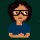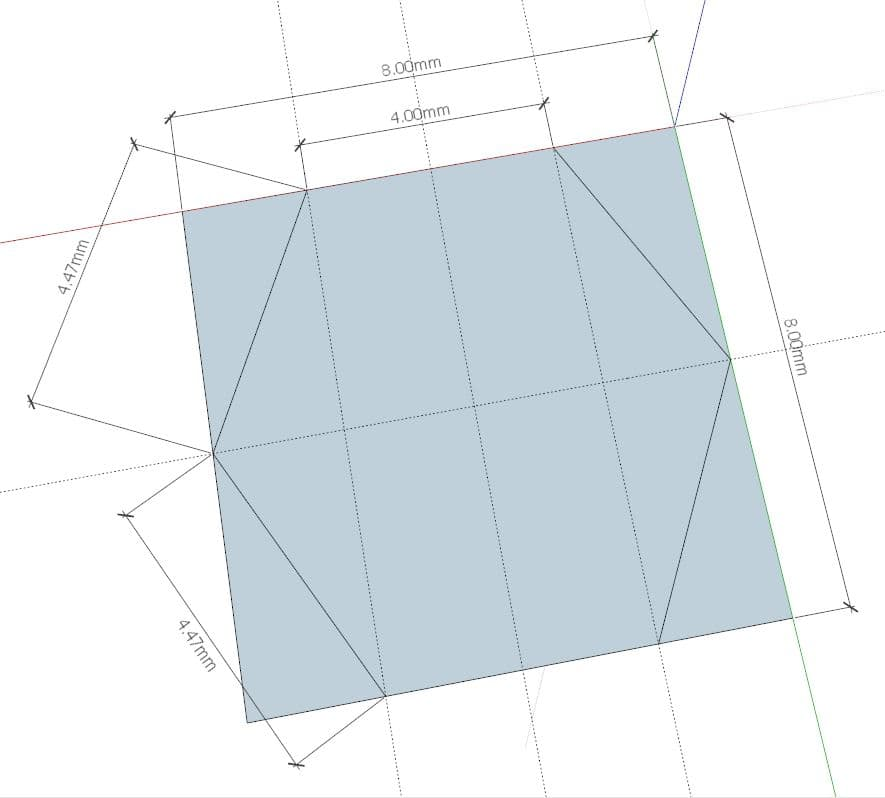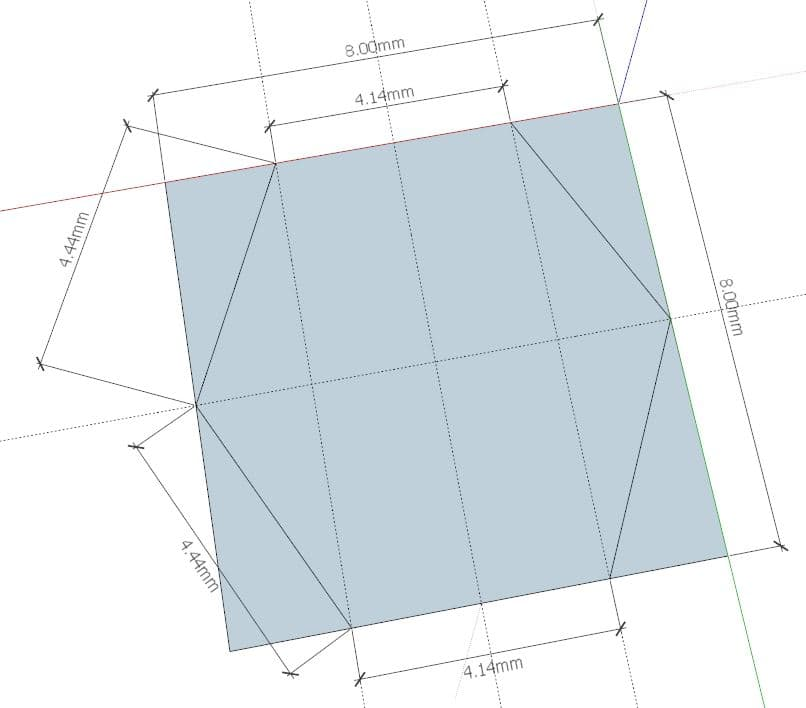Coffee Room
Discuss anything here - everything that you wish to discuss with fellow engineers.
12914 Members
Join this group to post and comment.Kaustubh Katdare • Dec 15, 2008

# Hexagon in a square

Here's an easier one for you -

What is the size of the largest regular hexagon that can be constructed inside a square with side-length 's'?

Crack it fast, please.shalini_goel14 • Dec 16, 2008
Hexagon side will be half of the side of square. i.e s/2 .Am I right ??i think it should be s/3....pls contradictshalini_goel14 • Dec 16, 2008
So easy to contradict over my answer.It was asked largest regular hexa gon so i think hexagon with side s/2 > s/3 .Am i right??Kaustubh Katdare • Dec 16, 2008
How'd we know if your answer is right or wrong? You have to provide an explanation along with your answer.durga ch • Dec 16, 2008
assuming the side of the regulr polygon to be x
assuming the size of the square to be a

any corner of the hexagon should not be concurrent with the corner of the square ,, as angle =90 which is not in a case of regular hexagon

hence working upon the right agnle trigaingle at one corner of the square which cannot be the part of the hexagon
since the polygon is aregular hexagon .. the internal angles are 120 degrees
thus considering one of the rigth corner tirangles
cos 60=((a-x)/2 )/x
x/2=(a-x)/2
x=a/2
now since x is the base of 6 equilateral triangles formed within the hexagon
the altitue being sqrt(3)/2*a/2=(sqrt(3)*a)/4
area of any triangle =1/2*b*h
1/2* (a/2) *((sqrt(3)*a)/4)
(sqrt(3)a^2)/16
since 6 such triangles are formed
6*(sqrt(3)a^2)/16

:rolling eyes:

i guess there is something i miseed.. its 1"30 AM here , so relatively no fuctionality of brianVidhya24 • Dec 16, 2008
X cannot be a/2...Kaustubh Katdare • Dec 16, 2008
This is why I love this section 😉 !

Don't look at me for the right answer fellas. When you are sure, you know you've gotten the right answer.durga ch • Dec 16, 2008
I dotn say I am correct, But I need a proof to say I am wrong...
I guess I can prove x=a/2
length os side of sq =a
max length of side of hexagon =a
since internal angle =120 degress
consider a point a on the side of the sq.. where the corner of a hexagon meets the sq side
internal angles -120 degrees
so sum of two external angles =60 degress
with the congruency of trinagles.. it can be proved that each extranl angle =30 degrees
and
as well....
the side oft he hexagon coincides with that of the side of sq
ie 180-120 degress is internal angle of the rt angle trinagle at the corner of the sq..

hence cos 60 =((a-x)/2)/x
ie x=a/2Vidhya24 • Dec 16, 2008
But try constructing it. U've considered a side of the square which coincides with a side of the hexagon. Now consider any two sides whose common edge lies on one of the sides of the square( but these two sides of hexagon do not lie on a side of the square).

So now, if x=a/2, these tho sides of the hexagon would lie on the side of the square... Think about it and contradict me if i'm wrong.durga ch • Dec 16, 2008
hmmm 😀

I understadn what you are saying...

I guess thats the same point 'a' what i talked about in the previous psot..

i mean even if two sides dont lie on one side of sq.. and opp two sides also dont lie on the sq...

you still ahve two side which can( and need) to lie on the sq...

Vidya..

may be you can try uplaoding an image if what you are trying to depict...

what if finally we both land up doing the same analysis!!! 😁Vidhya24 • Dec 16, 2008
In one of your previous posts, you have mentioned 'a' as a side, not a point...shalini_goel14 • Dec 16, 2008
Hey, i am changing my answer.My answer is s/sqrt(3). I will give explanation with figure.You need to wait for that.But you can tell either if it is right or wrong 😒 .silverscorpion • Dec 17, 2008
The area of the hexagon will be maximum when the diagonal of the hexagon is equal to the side of the square.

ie, if x is the side of the hexagon, then 2x is it's diagonal. So,

2x=a
x=a/2.

so the largest hexagon that can fit into a square of length a is of side a/2.
It must be oriented in such a way that two of it's sides coincide with the opposite sides of the square and two corners of the hexagon coincide with the midpoints of the other two sides of the square.

I hope I'm clear...well i think someone shud draw both s/2 and s/3 figures and post it....well i am sticking to my ansdurga ch • Dec 17, 2008
shalini_goel14
Hey, i am changing my answer.My answer is s/sqrt(3). I will give explanation with figure.You need to wait for that.But you can tell either if it is right or wrong 😒 .
hehe!!!😀
we have all very unique answers... no one can say what they have said is 100% the answer..

lets wait for some more entires😒Naveen89 • Dec 20, 2008
If the side of the square is s, then the side of hexagon would be
s/(2+√2)
. Considering the hexagon made would be regularNaveen89 • Dec 20, 2008
If the side of the square is s, then the side of hexagon would be
s/(2+√2)
. Considering the hexagon made would be regular.show with diagram!!!Naveen89 • Dec 21, 2008
Note: For Image see my avatar ( I dont know how to add image)
Y is the side of regular polygon (in red)
And x will be s-y;
X=y/√2 since the white portion forms a right triangle.
And x+y=s (given)
Putting the values
(y/√2)+y=s
Or y=√2s/(1+√2)Kaustubh Katdare • Dec 21, 2008
Naveen89
Note: For Image see my avatar ( I dont know how to add image)
Y is the side of regular polygon (in red)
And x will be s-y;
X=y/√2 since the white portion forms a right triangle.
And x+y=s (given)
Putting the values
(y/√2)+y=s
Or y=s/(1+√2)
Adding image is simple. Upload your image to Flicker.com - Get the best promotions on web are here. and then, copy the complete image path [obtained by right clicking on the image and selecting 'Copy Image Location' option] in the dialog box presented once you clickbutton in CE post editorThe_Big_K
Adding image is simple. Upload your image to Flicker.com - Get the best promotions on web are here. and then, copy the complete image path [obtained by right clicking on the image and selecting 'Copy Image Location' option] in the dialog box presented once you clickbutton in CE post editor
*psst* Flickr does not allow hotlinking 😉Kaustubh Katdare • Dec 21, 2008
ash
*psst* Flickr does not allow hotlinking 😉
[off-topic]
It does. All our images are hosted there 😒.

Here's an example -[CE's 3[sup]rd[/sup] Birthday Cake]I know it does. But I stress "not allow" as in policy wise 😉Naveen89 • Dec 21, 2008
but was my answer right?Kaustubh Katdare • Dec 21, 2008
How about " sqrt(2-sqrt(3)) s " or ~ 0.518 s?

---------------------------------------------------

@ Ash - I think the Flickr TOS says a 'visible link' should be provided along with the image. I'll remember that. Thanks 😀Jim Wrenholt • Jun 27, 2012
This is an old thread, and there was already a correct answer. But, it is a nice problem and the previous discussion left me unsure who was correct and why? So, with a little scratching...

If the hexagon has a radius of R and aligned so that a diameter of the hexagon is parallel to the bottom side of the square, then the correct answer is R = S/2. But if you rotate the hexagon a little you will be able to get a slightly larger polygon. By symmetry if you rotate the hexagon by 60 degrees you're right back where you started. If you rotate it 30 degrees you will have a diameter that is now perpendicular to the bottom side and you are still touching the square at R = S/2. It turns out that rotating it by 15 degrees will give you the maximum hexagon. So the correct answer is R = (S/2)/Cos(15) ~ 0.518*S.

You can use the fact that the Cos(15) = Cos(45 - 30) and the formula for the Cos(A - B) = Cos(45)Cos(30) + Sin(45)Sin(30) and much simplification to get the Sqrt(2 - Sqrt(3)) * S as already posted by The_Big_K.Gary Truesdale • Dec 11, 2016Gary Truesdale • Dec 11, 2016
Gary TruesdaleThis is a/2 in sketchup. Not quite right.Gary Truesdale • Dec 11, 2016
.518S is sill not quite right.Shashank Moghe • Dec 16, 2016
This might be previously answered based on intuition (drawing a hexagon inside a square) and I have to say that the intuition of drawing a hexagon - with its vertices on the sides of the square is wrong (I spent a lot of time doing the same, then realized the trigonometry does not give me the correct result - something very basic must be wrong). You can only draw such a polygon (n sides) for cases where n is a proper multiple (1,2,3..) of 4 (since for a square, n = 4). For a hexagon, such a sketch will bring us to wrong conclusions. And that is to say that the maximum sized hexagon inside the square will be tilted (no sides of the hexagon will coincide with that of the square). Please correct me if you find something wrong.

Therefore, this becomes a problem of optimization. I immediately thought about the Brachistochrone Problem. This problem literally led to the birth of a branch of mathematics called the Calculus of Variations.

I have grown to be increasingly drawn to this offshoot of calculus, since it was very recently that I learned about the Brachistochrone Problem. Now with this post, I started wondering if this optimization problem could be solved using calculus of variations. I understood the basics (very basic concepts) of the theory (that I learned online, a lot of literature is available), but the point where I am stuck is: how to find the "functional" to be optimized in this case.

During the course of our education, we learn to find the optima of a function (the procedure of finding the first derivative and equating it to 0, afterwards finding the second derivative and deciding if it is a local minima/maxima - is based on the calculus of variations. I did not know that.). It is easy if one is given the textbook problems, with boundary conditions. Here, I am thinking about the possible boundary conditions. A lot of unknowns.

I will continue to fight with this problem. Thus far, this thread has been highly rewarding.